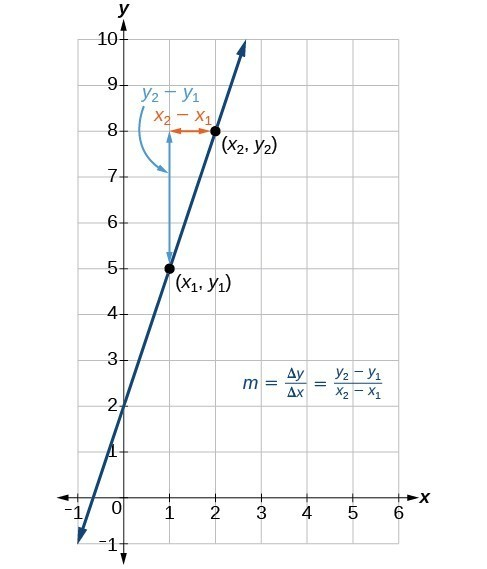## Calculate and Interpret Slope

### Learning Outcomes

• Define slope for a linear function
• Calculate slope given two points

One well known form for writing linear functions is known as slope-intercept form, where $x$ is the input value, $m$ is the rate of change or slope, and $b$ is the initial value of the dependant variable.

$\begin{array}{cc}\text{Equation form}\hfill & y=mx+b\hfill \\ \text{Function notation}\hfill & f\left(x\right)=mx+b\hfill \end{array}$

We often need to calculate the slope given input and output values. Given two values for the input, ${x}_{1}$ and ${x}_{2}$, and two corresponding values for the output, ${y}_{1}$ and ${y}_{2}$ —which can be represented by a set of points, $\left({x}_{1}\text{, }{y}_{1}\right)$ and $\left({x}_{2}\text{, }{y}_{2}\right)$—we can calculate the slope $m$, as follows

$m=\dfrac{\text{change in output (rise)}}{\text{change in input (run)}}=\dfrac{\Delta y}{\Delta x}=\dfrac{{y}_{2}-{y}_{1}}{{x}_{2}-{x}_{1}}$

where $\Delta y$ is the vertical displacement and $\Delta x$ is the horizontal displacement. Note in function notation two corresponding values for the output ${y}_{1}$ and ${y}_{2}$ for the function $f$ are ${y}_{1}=f\left({x}_{1}\right)$ and ${y}_{2}=f\left({x}_{2}\right)$, so we could equivalently write

$m=\dfrac{f\left({x}_{2}\right)-f\left({x}_{1}\right)}{{x}_{2}-{x}_{1}}$

The graph below indicates how the slope of the line between the points, $\left({x}_{1,}{y}_{1}\right)$ and $\left({x}_{2,}{y}_{2}\right)$ is calculated. Recall that the slope measures steepness. The greater the absolute value of the slope, the steeper the line is.The slope of a function is calculated by the change in $y$ divided by the change in $x$. It does not matter which coordinate is used as the $\left({x}_{2,\text{ }}{y}_{2}\right)$ and which is the $\left({x}_{1},\text{ }{y}_{1}\right)$, as long as each calculation is started with the elements from the same coordinate pair.

The units for slope are always $\dfrac{\text{units for the output}}{\text{units for the input}}$. Think of the units as the change of output value for each unit of change in input value. An example of slope could be miles per hour or dollars per day. Notice the units appear as a ratio of units for the output per units for the input.

### Calculating Slope

The slope, or rate of change, of a function $m$ can be calculated using the following formula:

$m=\dfrac{\text{change in output (rise)}}{\text{change in input (run)}}=\dfrac{\Delta y}{\Delta x}=\dfrac{{y}_{2}-{y}_{1}}{{x}_{2}-{x}_{1}}$

where ${x}_{1}$ and ${x}_{2}$ are input values, ${y}_{1}$ and ${y}_{2}$ are output values.

When the slope of a linear function is positive, the line is moving in an uphill direction from left to right across the coordinate axes. This is also called an increasing linear function. Likewise, a decreasing linear function is one whose slope is negative. The graph of a decreasing linear function is a line moving in a downhill direction from left to right across the coordinate axes.

In mathematical terms,

For a linear function $f(x)=mx+b$, if $m>0$, then $f(x)$ is an increasing function.

For a linear function $f(x)=mx+b$, if $m<0$, then $f(x)$ is a decreasing function.

For a linear function $f(x)=mx+b$, if $m=0$, then $f(x)$ is a constant function. Sometimes we say this is neither increasing nor decreasing.

In the following example, we will first find the slope of a linear function through two points then determine whether the line is increasing, decreasing, or neither.

### Example

If $f\left(x\right)$ is a linear function and $\left(3,-2\right)$ and $\left(8,1\right)$ are points on the line, find the slope. Is this function increasing or decreasing?

In the following video we show examples of how to find the slope of a line passing through two points and then determine whether the line is increasing, decreasing or neither.

### Example

The population of a city increased from $23,400$ to $27,800$ between $2008$ and $2012$. Find the change of population per year if we assume the change was constant from $2008$ to $2012$.

In the next video, we show an example where we determine the increase in cost for producing solar panels given two data points.

The following video provides an example of how to write a function that will give the cost in dollars for a given number of credit hours taken, x.